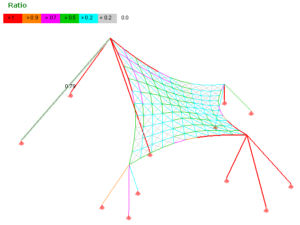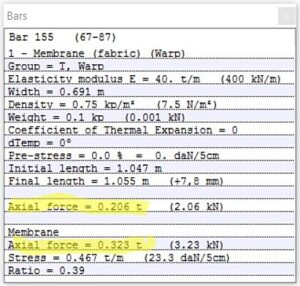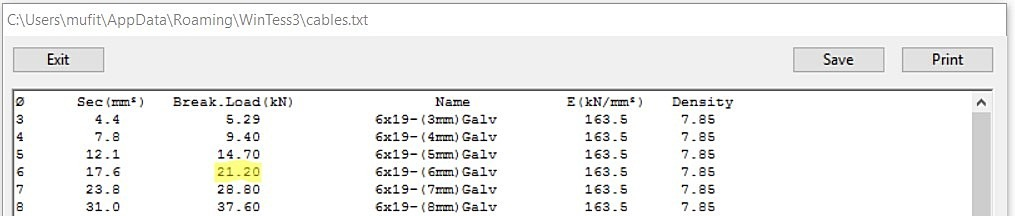##### Checking resultsOnce the calculation for the structure is performed, we can check if the selected components (membrane, cables, pipes, etc.) are correct. That is to say, if its resistance and behavior is acceptable.
WinTess performs this operation, and displays the results by the button Ratio on the left or the menu Graphics | Ratio.

##### Membrane type barsMembrane type bars have a certain axial force, as if it were a mesh of cables. But being a membrane, this force is not that we are interested in. What we really want to know is: what is the force on the membrane in the direction of the bar. This force tends to be higher especially in square mesh with diagonals, because that just adds the load applied on these diagonals. These values can be seen in the information box that can be accessed by clicking on a bar as seen in the figure on the right.

Tension in the membrane = Axial force membrane / Width of bar

The width of the bar is area of the membrane that has an impact on this bar (in general the distance between parallel bars in the same direction as the bar)

The ratio of the membrane type bar will be:

Ratio = Tension membrane x Factor of safety of the membrane / Membrane resistance

Keep in mind that WinTess supports a different resistance in the direction of the warp and the weft of the membrane. The diagonals the program takes an intermediate value between the resistances of warp and weft.

##### Cable type bars

The cable type bar can only withstand pulling. That is why the analysis of behavior is more simple. To do this we need to know only the details of the bar in question and the cable, and these are found in the database:We can get this as we know the breaking load of the cable. Thus the ratio of a cable will be:

Ratio = Load on the bar x Safety Coefficient of the cable / Breaking Load of the cable

In the cable type bar, if it is in a horizontal or tilted position and is long or heavy, we cannot forget the traction formed by the self weight of the cable. The best thing to do is to divide the cable into several bars and the program itself will take into account this value.

##### Tube type bars

Tube-type bars can withstand tensions and compressions.

The process is very similar to the previous cables for tension. The difference is that, in this case, we have no breaking load but elastic limit. The cross section area and section modulus of the tube are calculated from the diameter Ø and the thickness t. The elastic limit is obtained from the value S… that we have in the database.

Also we will have, at times, a stress due to bending by self weight of the tube . This is especially important for very thick tubes and quite inclined or horizontal position.Thus for a tube made of S235, the elastic limit is 235 N/mm2. With all this, we must:

Stress on the tube = Load on the bar / Cross section area of the tube + Moment for self weight / Section modulus

Ratio = Stress on the tube x Safety factor of the tube / Elastic limit

For the compression, the process is a little more complicated since it involves concept of buckling. Taking into account that WinTess is a program of second order, there is always the possibility of dividing the tube into many rigid bars articulated only at the ends of the tube and calculating that way. But this is only necessary in some particular cases. In general we will continue the following process:

WinTess calculates a reduction coefficient by buckling ψ, a value depending on λ, slenderness of the bar and its elastic limit. Then:

Stress on the tube =  Load on the bar  / (Cross section area of the tube x ψ )   +  Moment for self weight / Section modulus of the tube

Ratio =  Stress on the tube  x Safety factor of the tube / Elastic limit

##### Rigid bars

The rigid bars are a little more complex, but in the background is much like the previous case of the tubes. The most important difference is that in addition to the self weight, bending moment produced by the loads on the rigid bar  must be added. And this time, it is usually much greater than that of own weight. In addition, there is a tension produced by the shear stress, although in most cases this stress is quite insignificant in the whole of tensions (found in another plane and we must resort to the stress of von Mises to combine them).

Stress on rigid bar =  Load on the bar  / (Cross section of the tube x ψ )   +  (Bending moment loads + Moment for self weight) / Section modulus of the tube

Ratio =  Stress on rigid bar  x Safety factor of the tube / Elastic limit